# Brainteaser Answers – Issue 40, 2018

Did you figure out this issue’s answers? If not, we’re here to help! Check out the solutions below.

## 1.) Loopy Lapel

Solution:

There are many YouTube videos that show the setup and solution, here is a good one: https://www.youtube.com/watch?v=MrN1hNzXoec

Find all real numbers x such that: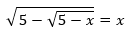Solution:

Square both sides (just remember that this process may give extraneous solutions – we will test each for validity later) to get: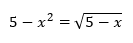Square again to get:

Or:

##### (x2 + x – 5)(x2 – x – 4) = 0

Setting the second term (x2 – x – 4)  to zero gives: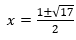but neither answer satisfies the original equation.

Setting the first term (x2 + x -5) to zero yields: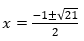but only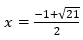is a valid solution.  And therefore it is the answer.

## 3) Alphametics

Solution:

Since each letter in the alphametic represents a unique and different “digit” and there are eight different letters used (O, N, E, T, W, F, U, R), the positive integral base must be equal to, or greater than, eight.Some “truths” we can determine without regard for the base:

• O + O = R, but O + O = T; therefore, in ONE+ ONE= TWO, a 1 must be carried over from N + N.
• Therefore 2O = R, 2O + I = T, R + l = T, R is even, T is odd, and F = I.
• If O = zero, then R = zero. Therefore  O is neither 0 nor 1.
• Also, O must be less than one-half of the base, since 1 + O + O = T does not exceed the base, and O + 0 = R does not exceed the base.

Now let’s label the base b.

1 < O < b/2.

• If b = 8, O = 2 or O = 3. (Neither works.)
• If b = 9, O = 2, O = 3, or O = 4. (None of these works.)
• If b = 10, then O = 2, O = 3, or O = 4. (None of these checks).
• If b = 11 or b = 12, then O = 2, O = 3, O = 4, or O = 5. (None of these checks in either base.)
• If b = 13, O = 2, O = 3, O = 4, O = 5, or O = 6. (None of these checks in base 13.)
• If b = 14, O = 2, O = 3, O = 4, O = 5, or O = 6.

If the digits of base 14 are {1, 2, 3, 4, 5, 6, 7, 8, 9, tn, el, tw, th}

Then when O = 4, a solution is found:

ONE + ONE = TWO – 4(tn)2 + 4(tn)2 = 964,

TWO + TWO = FOUR – 964 + 964 = 14(tw)8.

Therefore, O = 4, N = tn, E = 2, T = 9, W = 6, F = 1, U = tw, and R = 8.

Thus, the smallest base for which these alphametics hold true is 14.

[credit: Mathematics Student Journal – circa 1965]